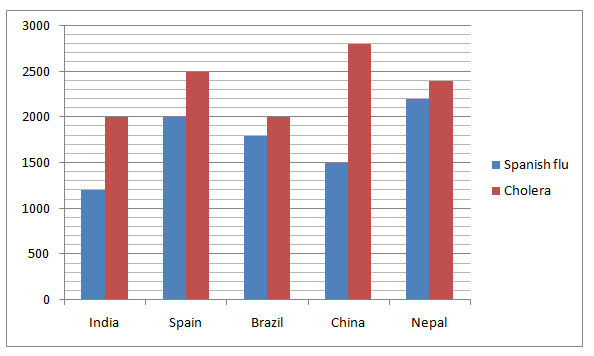# IBPS PO Prelims Quantitative Aptitude Questions 2021 (Day-07)

Dear Aspirants, Our IBPS Guide team is providing new series of Quantitative Aptitude Questions for IBPS PO Prelims 2021 so the aspirants can practice it on a daily basis. These questions are framed by our skilled experts after understanding your needs thoroughly. Aspirants can practice these new series questions daily to familiarize with the exact exam pattern and make your preparation effective.

Start Quiz

Bar graph

Directions (1-5): Study the following information carefully and answer the questions given below.

The given bar graph shows the number of people died on two different diseases – Spanish flu and Cholera in five different countries.1) What is the average number of people died of Cholera in all the countries together?

A.2340

B.2450

C.2350

D.2430

E.2530

2) The number of people died on Spanish flu in Nepal is approximately what percent of the number of people died on both diseases in Brazil?

A.55%

B.58%

C.45%

D.40%

E.63%

3) The number of people died on Spanish flu in Bangladesh is 80% of the people died on Cholera in China and the number of people died on Cholera in Bangladesh is double of the number of people died on cholera in India. What is the difference between the number of people died on Spanish flu and Cholera in Bangladesh?

A.1750

B.1740

C.1780

D.1760

E.1770

4) What is the ratio of the number of people died on both diseases in India to number of people died on both diseases in Spain?

A.30:43

B.31:44

C.32:45

D.33:46

E.None of these

5) What is the difference between the number of people died on Spanish flu in China and the number of people died on Cholera in Nepal?

A.800

B.700

C.500

D.1000

E.None of these

Directions (06-09): Following question contains two equations as I and II. You have to solve both equations and determine the relationship between them and give answer as,

6) I) X2-12X+35=0

II) 2Y-11Y+14=0

A.X > Y

B.X ≥ Y

C.X = Y or relationship can’t be determined

D.X < Y

E.X ≤ Y

7) I)
X2-39X+74=0

II) Y2-18Y+17=0

A.X > Y

B.X ≥ Y

C.X = Y or relationship can’t be determined

D.X < Y

E.X ≤ Y

8) I)
X=√324 +  37

II) Y=√(324+37)

A.X > Y

B.X ≥ Y

C.X = Y or relationship can’t be determined

D.X < Y

E.X ≤ Y

9) I)
X2+16X+48=0

II) Y2-14Y+33=0

A.X > Y

B.X ≥ Y

C.X = Y or relationship can’t be determined

D.X < Y

E.X ≤ Y

Time and work

10) The efficiency of A is 200% more than the efficiency of C and the efficiency of D is 25% less than the efficiency of C. If A and B together can complete the work in 14 days and C and D together can complete the work in 36 days, in how many days B alone complete the work?

A.42 days

B.45 days

C.48 days

D.40 days

E.39 days

### Try IBPS PO Prelims Free Online Mock Test

Required average = (2000 + 2500 + 2000 + 2800 + 2400)/5

= 2340

Required percentage = 2200/(1800 + 2000) * 100

= 58%

Number of people died on Spanish flu in Bangladesh = 80/100 * 2800 = 2240

Number of people died on Cholera in Bangladesh = 2 * 2000 = 4000

Difference = 4000 – 2240 = 1760

Required ratio = (1200 + 2000):(2000 + 2500)

= 32:45

Difference = 2400 – 1500 = 900

X2 -12X +35 = 0

X2-7X -5X+35=0

X(X-7)-5(X-7)=0

(X-7)(X-5)=0

X=7,X=5

2Y-11Y +14 =0

2Y2-7Y-4Y+14=0

Y(2Y-7)-2(2Y-7)=0

(Y-2)(2Y-7) =0

Y=2, Y= 7/2

X > Y

X2 -39X +74 = 0

X2-37X -2X+74=0

X(X-37)-2(X-37)=0

(X-37)(X-2)=0

X=37,X=2

Y-18Y +17 =0

Y2-17Y-1Y+17=0

Y(Y-17)-1(Y-17)=0

(Y-17)(Y -1) = 0

Y=17,Y=1

Relationship cannot be established

X=18+37  = 55

Y=√361

Y=19

X > Y

X2 +16X +48 = 0

X2+12X +4X+48=0

X(X+12)+4(X+12)=0

(X+4)(X+12) = 0

X=-4,X=-12

Y-14Y +33 =0

Y2-11Y-3Y+33=0

Y(Y-11)-3(Y-11)=0

(Y-11)(Y-3)=0

Y=11,Y=3

X < Y

Efficiency of A and C = 300:100 = 3:1

Time ratio of A and C = 1:3

Efficiency of C and D = 100:75 = 4:3

Time ratio of C and D = 3:4

1/3x + 1/4x = 1/36

7/12x = 1/36

x = 21

C alone complete the work = 3 * 21 = 63 days

A alone complete the work = 1/3 * 63 = 21 days

B alone complete the work = 1/14 – 1/21 = 1/42

For More Days IBPS PO Prelims Practice Set 2021

Get Quantitative Aptitude Study Materials

Get All Section Study Materials

5 1 vote
Rating